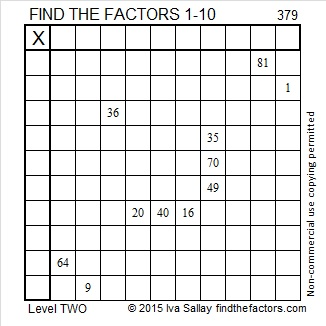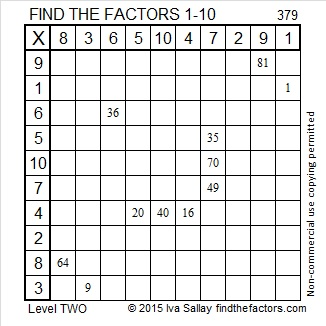# 379 and Level 2

2^379 (mod 379) = 2 so 379 is VERY LIKELY a prime number. Scroll down below the puzzle to know for sure.

Alan Parr, who worked on some fun projects at NRICH, recently wrote about his game, Envelopes, on his blog. This easy-to-make game can be used to reinforce addition or multiplication facts, and it never looks anything like a worksheet.Print the puzzles or type the factors on this excel file: 10 Factors 2015-02-02

• 379 is a prime number.
• Prime factorization: 379 is prime.
• The exponent of prime number 379 is 1. Adding 1 to that exponent we get (1 + 1) = 2. Therefore 379 has exactly 2 factors.
• Factors of 379: 1, 379
• Factor pairs: 379 = 1 x 379
• 379 has no square factors that allow its square root to be simplified. √379 ≈ 19.468How do we know that 379 is a prime number? If 379 were not a prime number, then it would be divisible by at least one prime number less than or equal to √379 ≈ 19.468. Since 379 cannot be divided evenly by 2, 3, 5, 7, 11, 13, 17, or 19, we know that 379 is a prime number.This site uses Akismet to reduce spam. Learn how your comment data is processed.